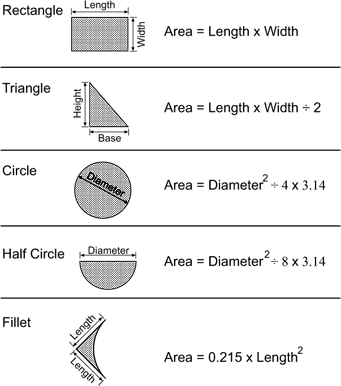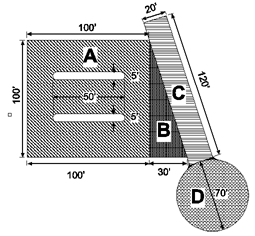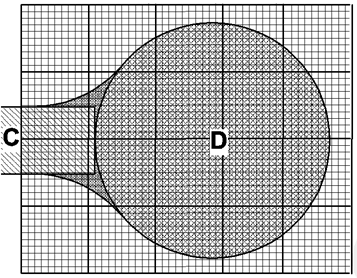How to Determine Quantities

# How to Determine QuantitiesTo determine the amount of asphalt you will need for a paving job you first need to know the area to be paved. This may be simple if the area to be paved is square, rectangular or constant width of road. However, this is seldom the case since there may be planters in the area to be paved, the width of the road may vary or there are cul-de-sacs.
This may seem to be impossible in some cases, but by dividing the pavement area into simple geometric shapes and adding and subtracting these areas the area to be paved can be quickly determined. The following are basic formulas for calculating the area of different geometric shapes.
Lets look at the parking lot in Figure 1, since they often have many features that complicate the calculation of area.
When you first look at this it may seem complicated since it is not a square, triangle or circle. However, it is a combination of squares triangles and circles as can be seen in Figure 2.By dividing a project into these simple shapes you can now easily calculate the area to be paved.
Area A is a rectangle 100’×100′ = 10,000 square feet
Area B is a triangle 30’×100’÷2 = 1,500 square feet
Area C is a rectangle 20’×120′ = 2,400 square feet
Area D is a circle 702 ÷ 4 ×3.14 = (70×70) ÷ 4 ×3.14 = 3,846.5 square feet.
To determine the total area of paving all we have left to do is add the areas together with one exception, the planters in the middle of the parking area. All we have to do here is calculate the area of the planters and subtract that area from the sum of the other areas. But I’m tired now so I will leave the calculation of the planter areas up to you. Just remember the planter areas are just rectangles with semi-circles at each end.
Therefore, the total paved area is:
10,000 + 1,500 + 2400 +3846.5 – (Planter Area) = 17,257 square feet.
OK, I did calculate the planter area, I just wanted you to have a try at this. I also rounded off to the nearest whole number. This is all the accuracy you will likely ever need on a paving job.
You may notice that there are small areas around the curb returns at the cul-de-sac that were not calculated. In this case the areas are small and will not make a significant difference in the total areas. If you have many areas like this on a project it would probably be best to draw the area to scale on graph paper and estimate the area by counting the number of squares and multiplying the number of squares by the area of each square. For example if you drew the area at a scale of 1″=20′ and the graph paper was divided into 0.10″ increments each square would have an area of 4 square feet (2×2). If you counted 20 squares, the total area would be 80 square feet (20×4).
Figure 3 illustrates an example of this. In this case there are approximately 20 squares. One of the tricks is to combine partial squares. For example if 3/4 of one square is covered by the area and 1/4 of another square is also covered by the area they can be combined and counted as a full square.Now that you have the area calculated, you next need to calculate the quantities. Quantities are usually specified by weight (tons) or volume (cubic yards). No matter how it is specified you will first need to calculate the volume. Volume is easy to calculate since it is simply Area × Thickness. One of the keys in calculating volume is to always use the same dimension. Therefore, if we measure the area in square feet we have to convert thickness measurement to feet also. For example if you are placing 6″ of aggregate base the thickness would be converted to:
6″÷12″/foot = 0.5 feet.
To compute the volume we simply multiply this thickness by the area previously computed.
Volume = Area × Thickness = (17,257 square ft.) × (0.5 feet) = 8628.5 cubic ft.
Aggregate base and other material are often specified or purchased by the cubic yard; therefore, you need to convert cubic feet to cubic yards. Since there are 27 cubic feet per cubic yard (3’/yard × 3’/yard × 3’/yard) you would need to divide the cubic foot volume by 27.
Volume = 8628.5 cubic ft. ÷ 27 cubic ft/cubic yd. = 320 cubic yds.
To calculate the weight of material needed for a project you will need to know the compacted density of the material. Density is the weight of material per volume. For example pounds per cubic foot. The density of Hot Mix Asphalt (HMA) does vary depending on mix proportions and type of aggregate. If you do not know the in-place density of mixes you use ask your supplier.
To determine the weight of material multiply the volume by the density of material:
Weight = Volume × Density
Using the previous example and assuming an HMA density of 145 pounds per cubic foot (pcf) and a thickness of 4″ we would perform the following calculations.
1         Volume = 17,257 sq. ft.× (4″÷12″/ft) = 5,752 cu. ft.
(notice that the thickness was converted from inches to feet)
2         Weight = 5,752 cu. ft. × 145 pcf = 834,040 lbs.
3         Convert to tons = 834,040 lbs. ÷ 2,000 lbs/ton = 417 tons

Now it’s your turn to try your new skills. Using the paving area in Figure 2, calculate the cubic yards of aggregate base and tons of HMA needed assuming the following.

1         Areas A & B are to be paved using 4″ of Aggregate Base and 3″ of HMA.)
2         Areas C & D are to be paved using 6″ of Aggregate Base and 4″ of HMA.

 Answers: Areas A & B: Agg. Base = 136 cu. yds; HMA = 200 tons Areas C & D: Agg. Base = 116 cu. yds: HMA = 151 tons